Climate Change in Australia

Climate information, projections, tools and data

Scaling Methods

Detailed explanation of the mean and quantile-quantile scaling methods used

Mean scaling

The essentials of the approach used for mean scaling are illustrated in Figure 1, below. For details of which variables have been scaled in this way, see the table of variables on the Application-ready data page.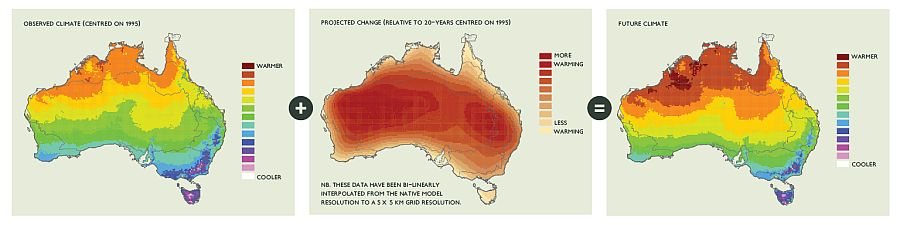Fig.1. An example of the ‘delta change' (or ‘perturbation') method using mean scaling. Here, future climate temperature information (right) is created by adding projected temperature change information from a climate model (middle) to observed data (left).

The steps for producing a mean scaled monthly time-series.

For each 5 x 5 km grid point:

1. Calculate historical GCM climatology (20-30 yrs) for each month.
2. Calculate future GCM climatology (20-30 yrs) for each month.
3. Calculate absolute change by subtracting future from historical for each month.
4. Calculate percent change by dividing abs change by the historical for each month.
5. For temperature related variables add the absolute change, for all others multiply by the percent change for each month, to data in each month of the observational time-series.

Production of future daily time-series uses the same approach but at the final step, the monthly change value applied is that for the month corresponding to the historic date. For example, in the example shown in Figure 2, a July daily value from the observed data is scaled by the projected change for July.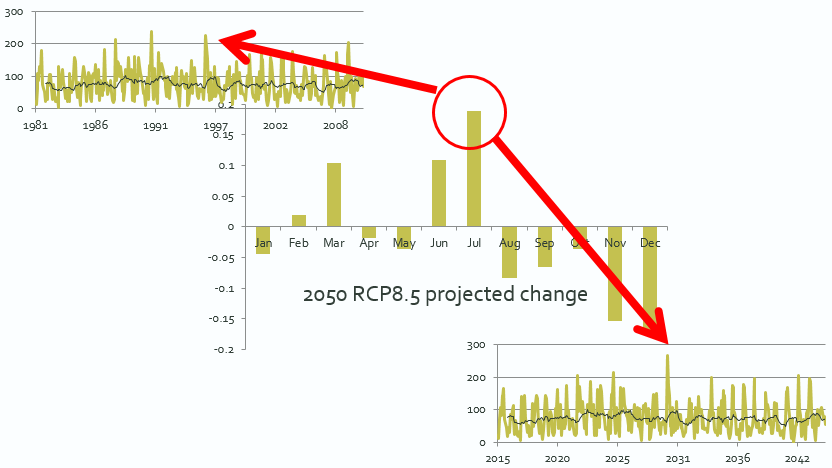Fig.2. An example showing a daily time-series (top left) being scaled by the appropriate monthly mean change (middle) to produce a plausible future daily time-series (bottom right). The arrows show a July day from the observations being scaled by the July change value to produce the corresponding future daily amount.

Quantile-quantile scaling

Quantile-quantile scaling is a modification to the delta change technique that captures important changes in daily variance (see the Technical Report ). For most climate variables, this is not a significant factor, but for daily rainfall it is. Climate models indicate that extreme daily rainfall intensity and frequency is likely to increase, even in areas where mean rainfall shows little change or a decrease. This represents an increase in daily variance. Capturing this expected change in extreme events in application-ready datasets is very important for future planning. Therefore, quantile-quantile scaling has been used in the production of future daily rainfall data. The quantile-quantile scaling method used for Climate Change in Australia is significantly more complex than mean scaling.

Step 1: Create quantile-quantile mapping functions for each month from the Global Climate Model (GCM) data (see Figure 3.)

For each 5 x 5 km grid point:

1. Rank GCM historical and future daily time-series values from smallest to largest.
2. Group ranked values into 10 decile bins, with decile 1 containing the lowest 10% and decile 10 the highest 10% of values.
3. To provide more detailed changes in extreme events, divide the 10th decile values into 10 percentile mapping bins, thus giving 19 quantiles (9 deciles plus 10 percentiles) in total.
4. Calculate mean values for each decile and upper percentile (quantiles) for both the historical and future data (see Figure 3, bottom left).
5. Calculate the change ratio for each quantile by subtracting the historical mean from the future and dividing by the historical (see Figure 3, right).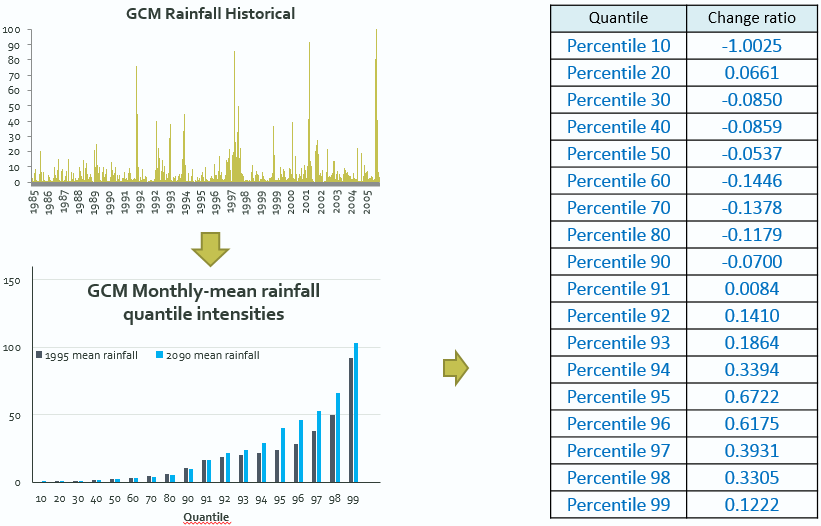Fig.3. Step 1 of quantile-quantile scaling. GCM simulated historic daily rainfall data (top left) are 'binned' into 19 quantiles (the 100th percentile is not shown). The same is done for the model's future projected times series (bottom left). The change from historic to future is calculated for each 'bin' or quantile (right).

Step 2: Modify Observed data (Figure 4)

For each 5 x 5 km grid point:

1. Rank observed daily time-series values from smallest to largest for each month.
2. Group ranked values into 10 decile bins, with decile 1 containing the lowest 10% and decile 10 the highest 10% of values.
3. Divide the 10th decile values into 10 percentile mapping bins (20 quantiles total).
4. Apply the GCM-based change ratios from each quantile level to all observational values in each quantile. (Multiply the mean change for the GCM quantile by the mean obs quantile value, then add to the obs value).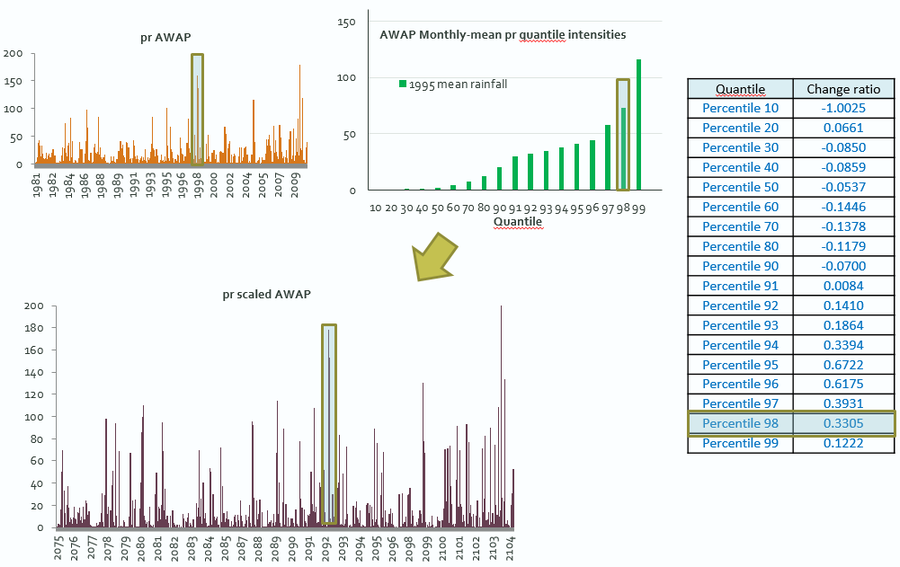Fig.4. Observed daily rainfall time-series (top left) 'binned' into quantiles (top middle). The quantiles are scaled by the corresponding change ratios (right) and applied to the observations to produce the scaled future time-series (bottom left).

Important notes regarding the time-series datasets

1. The observed data used are a 30-year time series (1981 to 2010).
2. The change values applied are calculated from 20-year time-slices where the historic baseline period is 1986 to 2005. The future time periods are: '2030' (2016 to 2045), '2050' (2036 to 2065), '2070' (2056 to 2085) and '2090' (2075 to 2104).
3. The future time-series produced are therefore 30-years in duration but representative of the projected mean climate state for that time.
4. For this reason, although the four future time-periods overlap slightly, they do not join (see Figure 5). It is not appropriate to try to use the separate time-series to build a plausible continuous time-series for the whole 21st Century.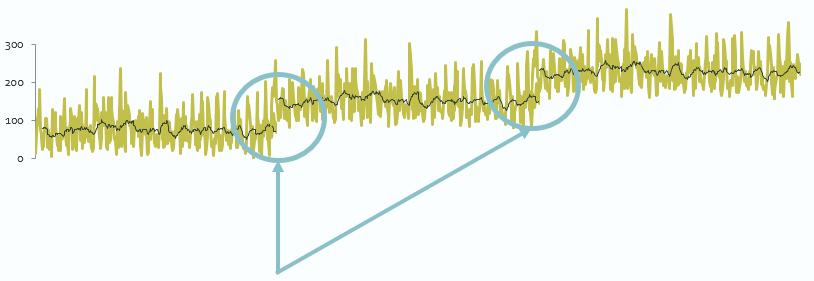Fig.5. Illustration of the discontinuity in consecutive future time-series datasets. Note that the actual time-series datasets overlap more than indicated.

Page updated: 13th July 2020